# Sand path

How many m3 of sand is needed to fill the 1.5m wide path around a rectangular flowerbed of 8m and 14m if the sand layer is 6cm high?

V =  4.5 m3

### Step-by-step explanation: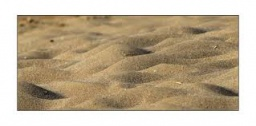Did you find an error or inaccuracy? Feel free to write us. Thank you!Tips to related online calculators
Do you want to convert area units?
Do you want to convert length units?
Do you know the volume and unit volume, and want to convert volume units?

#### You need to know the following knowledge to solve this word math problem:

We encourage you to watch this tutorial video on this math problem:

## Related math problems and questions:

• Round flowerbed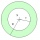Around a round flowerbed with a diameter of 6 meters and I will make a sidewalk up to 0.5 meters wide. How much gravel is needed if the layer is to be 5 cm high?
• Three-quarters of its volume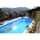The pool has a block shape with a length of 8m, a width of 5.3m and a depth of 1.5m. How many hl of water is in it if it is filled to three-quarters of its volume?
• Concrete boxThe concrete box with walls thick 7 cm has the following external dimensions: length 1.8 m, width 44 cm and height 46 cm. How many liters of soil can fit if I fill it to the brim?
• Pool 3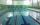How long will fill pool cuboid shape (8m 6m 1.5m) when flows 15 liters/s?
• Cuboid boxHow many m2 paper is needed for the sticking cuboid box of dimensions 50 cm, 40 cm and 30 cm? To the folds add one-tenth the area.
• SnowSnow fell overnight layer of thickness 19 cm. In the morning I had to clear a path 69 m long and one meter wide. How many cubic meters of snow I clear? How many kilos was it? (1 m3 fresh snow weighs 350 kg)
• TetrapackHow high should be the milk box in the shape of a prism with base dimensions 8 cm and 8.8 cm if its volume is 1 liter?
• Prism - boxThe base of prism is a rectangle with a side of 7.5 cm and 12.5 cm diagonal. The volume of the prism is V = 0.9 dm3. Calculate the surface of the prism.
• Square prismCalculate the volume of a square prism of high 2 dm wherein the base is: rectangle with sides 17 cm and 1.3 dm
• FlowerbedThe flowerbed has a length 3500mm and a width 1400mm. How many foil is needed to covers the flowerbed? How many m2 of foil was consumed for its production (add 10% of the material to the joint and waste)? How many liters of air is inside the enclosure? (F
• Sandbox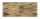How much cubic meters of sand is needed to fill the sandbox on the field if it has the shape of a circle with a diameter of 3 m and is 25 cm deep?
• PoolMr. Peter builds a pool shape of a four-sided prism with a rhombus base in the garden. Base edge length is 8 m, the distance of the opposite walls of the pool is 7 m. The estimated depth is 144 cm. How many hectoliters of water consume Mr. Peter to fill t
• Body diagonalCalculate the volume of a cuboid whose body diagonal u is equal to 6.1 cm. Rectangular base has dimensions of 3.2 cm and 2.4 cm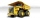The truck with a load capacity of 7 tons has a storage area of 4.5 m and 2.1 m. The weight of 1 m3 of wet sand is 2,000 kg. How high can we load the sand so that the load capacity of the car is not exceeded?On Thursday, fell 1 cm rainfall. How many liters of water fell to rectangular garden with dimensions of 22 m x 35 m?Calculate the volume of the bases of a square house, if the base depth is 1.2 m, the width is 40 cm and their outer circumference is 40.7 m.How many bricks are needed for a wall 14.5 m long, 2 m high and 45 cm thick, if 285 bricks are needed for one m ^ 3 wall?